# The Cube

The cube is probably the most recognized and best known of and 3D shape. Kids young enough not to even know how to talk will still know about cubes, they play with multicolored wooden blocks. Older kids may tackle the Rubik’s Cube puzzle or play Yahtzee with dice. Then they may graduate to working in a cubicle as adults.

We use the cube as a representation of the space around us. Pepsi was briefly sold in 24-can “cubes.” Apple’s G4 computer was cubic.

Al Kaaba (literal English, the cube) is a cuboid shaped building in Mecca and the most sacred site to Islam.

Salt grows into cubic crystals.

Stones chipped and shaped into cubes have been found that date back 100’s of thousands of years.

Star Trek’s fictional, I hope, Borg traveled through space in a cube shaped ship.

There are several prisons with the nickname “the cube,” despite not having walls as tall as they are wide.

They are literally, all around us. We have have such a familiarity with the cube that we don’t even have to think about its qualities or structure. We just know it.

Therefore, having a page devoted to the cube may seem like a waste of space, but I’m certain that there is still knowledge we can gain from re-examining the world around us.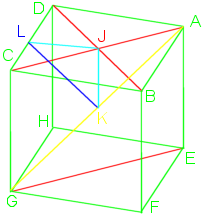The cube is a 3 dimensional solid object made of 6 squares (variously called faces, facets, or sides), each meeting at right (90°) angles with two others. It is one of the 5 Platonic solids and can also be referred to as a regular hexahedron or a square prism.

Like a sphere is the set of all points equidistant from the origin, the cube is the set of points that make up the square faces, it has no volume, although we usually refer to the volume it contains.

If we call the edge length S, then  each face will have area $$S^2$$, so the cube will have surface area $$6*S^2$$. The internal volume is $$S^3$$.

Many different shapes can tile 2D space, squares, hexagons, various triangles. The cube is the only object that can regularly tile Euclidean 3D space.

Using formulas already mentioned, vertex edge angles are: $$\Large\cos\mu=\frac{cos90}{cos45}=\frac{0}{\frac{1}{\sqrt2}}=0, \mu=90^\circ$$.

Using a cube with vertexes named A-H, as in the image, with edge length of 1, we can then calculate other lengths, easily done with right triangles and the Pythagorean theorem. Actually, it is even easier as almost all will be 45° right triangles.

Drawing a diagonal across a face from B to D, we get a right triangle with BD length $$\sqrt2$$. Drawing diagonal AC crosses at the midpoint J.

Drawing a spacial diagonal through the cube from A to G, triangle AEG lengths would be $$1,\sqrt2, and \sqrt3$$. Midpoint of AG is K which is also the spacial midpoint of the cube. AK and GK are $$\frac{\sqrt3}{2}$$, the radius of a sphere surrounding the cube.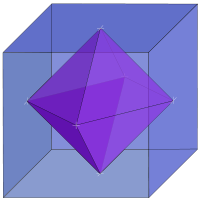To find JK:
\begin{align} (AJ)^2+(JK)^2&=(AK)^2\\ (JK)^2&=(AK)^2-(AJ)^2\\ &=\left(\frac{\sqrt3}{2}\right)^2-\left(\frac{\sqrt2}{2}\right)^2\\ &=\frac34-\frac24\\ &=\frac14\\ JK&=\frac12\end{align} so JK=$$\frac12$$. This is the radius of an inscribed sphere.

The midpoint of CD is L. Line JL would 1/2 the edge length, equal to JK, so $$KL=\frac{\sqrt2}{2}=\frac1{\sqrt2}$$. Angle JLK is 45°, one half the dihedral (face to face) angle.

If you join the mid-face points (all the J’s), you would then get the cube’s dual shape called the octahedron. Similarly, joining the midpoints of the octahedron’s face would give you a cube. An octahedron inside a cube of edge length S would have edges of length $$S\cdot\frac1{\sqrt2}$$ and an octahedron outside the cube would have edge length of $$S\cdot\sqrt2$$. The octahedra would have volumes of $$S^3\cdot\frac13$$ and $$S^3\cdot\frac23$$, respective.

# An unequal pyramid

Last post, I worked on the vertex edge angles of a triangular pyramid that had all equal angles originating from the apex.

Using the same camera tripod analogy, take one of the legs (line DA) of the tripod and slide it out further from the other two, but keep it equally distant from each. You now have a camera that’s about to crash to the floor, but you also have a pyramid that has two equal apex angles and that leans.

A right pyramid is a pyramid for which the apex lies directly above the centroid of the base. I don’t know if there is an actual name for a leaning pyramid, but I will just call it a leaning pyramid.

Again, we will work with a pyramid with vertexes A, B, C, & D, with D as the apex (Fig. 1). Again, the legs DA, DB, & DC are all equal length of 1 unit.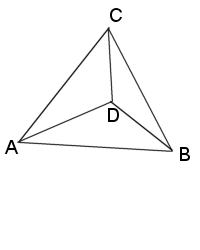Figure 1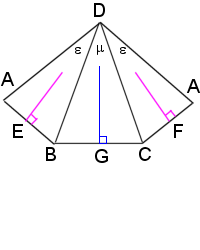Figure 2

We can “unfold” the pyramid (Fig. 2) to show the bisection of the apex angles where they join the base vertexes, the midpoints E, F, & G.

We can see BG=CG, and AE=BE=CF=AF, and DE=DF.

Since ΔDAB = ΔDAC, we will ignore showing the the latter in the formulas. Both are isosceles, as well as ΔDBC.

$$\large{ BG=CG= sin(\frac{\mu}{2})\\ BC=2\cdot sin(\frac{\mu}{2})\\ DG=cos(\frac{\mu}{2})\\ AE=BE= sin(\frac{\epsilon}{2})\\ AB=2\cdot sin(\frac{\epsilon}{2})\\ DE=cos(\frac{\epsilon}{2})}$$

Items that must be true for this to be a real 3D object: $$2\cdot\epsilon>\mu, 2\cdot\epsilon+\mu<360^\circ$$.

Figure 3 shows how to find the line AG on the base triangle. Using the Pythagorean theorem, $$AG=\sqrt{4\cdot sin^{2}(\epsilon/2)-sin^{2}(\mu/2)}$$.

We split the pyramid in half, giving us cross section ΔADG (Fig. 4).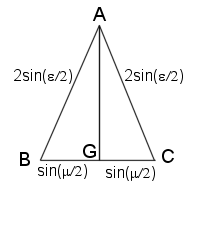Figure 3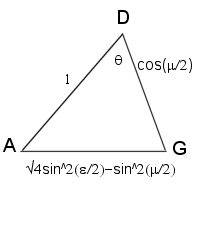Figure 4

Using law of cosines, we get:

\large{\begin{align}\cos\theta &=\frac{1^2+\cos^{2}(\frac{\mu}{2})-4\cdot \sin^{2}(\frac{\epsilon}{2})+\sin^{2}(\frac{\mu}{2})}{2\cdot 1\cdot \cos(\frac{\mu}{2})}\\ &=\frac{1 +[\cos^{2}(\frac{\mu}{2})+\sin^{2}(\frac{\mu}{2})]-4\cdot \sin^{2}(\frac{\epsilon}{2})}{2\cdot \cos(\frac{\mu}{2})} &\text{Rearrange figures}\\ &=\frac{1+-4\cdot \sin^{2}(\frac{\epsilon}{2})}{2\cdot \cos(\frac{\mu}{2})} &\text{cos²α + sin²α = 1}\\ &=\frac{2-4\cdot \sin^{2}(\frac{\epsilon}{2})}{2\cdot \cos(\frac{\mu}{2})}\\ &=\frac{1-2\cdot \sin^{2}(\frac{\epsilon}{2})}{\cos(\frac{\mu}{2})}\\ &=\frac{\cos(\epsilon)}{\cos(\frac{\mu}{2})} & \text{1-2sin²α = cos 2α} \end{align}}

We can now use this new formula to double check our work on the truncated icosahedron. Hexagons have exterior angles of 120° (ε) and pentagons are 108° (μ). So we get:

$$cos\theta=\frac{\cos(120)}{\cos(\frac{108}{2})}=\frac{cos(120)}{cos(54)}\\ \theta=148.2825256\ldots^\circ$$

The formula can be used to quickly find the vertex edge angle of any of the Platonic or Archimedean solids that have only 3 shapes meeting at each vertex, with 2 (or all 3) of them the same. (Although it isn’t as much fun as actually working it out ourselves.) It can also be used on prisms, but that is trivial, as the edge will always be at 90° to the bases.

We can find the angle made by slicing the pyramid through ΔCDE, but the result really doesn’t mean much as the plane made by ΔCDE is not perpendicular to the plane made by the base. ΔADG is perpendicular to the base plane. There would be 2 angles needed to describe the result. Example, looking at the truncated icosahedron, you can see the edges splaying out from the pentagons even with the center lines, sort of like the spokes of a wheel, but looking at the hexagons, the edges don’t line up with the center lines, they are slightly off.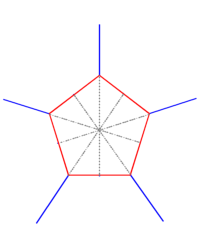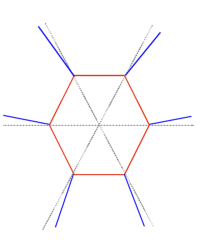Likewise, in a pyramid with all 3 apex angles different, there would be no cross section that was perpendicular to the base plane, so no real usage here.

# Tetrahedron

I started off posting about the truncated icosahedron, despite being fairly complex compared to the platonic solids, like the tetrahedron.

The regular tetrahedron is probably the simplest 3D shape, except for maybe the sphere. It is made of 4 equilateral triangles, forming a triangular pyramid.

Starting with a tetrahedron with vertexes named A, B, C, & D, with D as the apex (fig. 1), we can bisect ΔABC from point A to line BC and call that point G (fig. 2).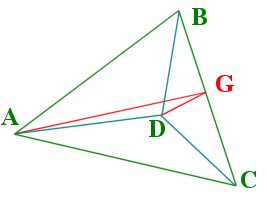Figure 1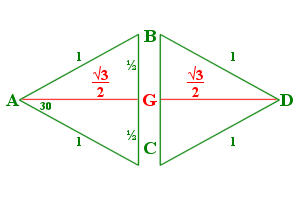Figure 2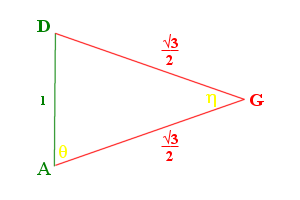Figure 3

The bisection of ΔDBC would follow the same, a line DG would also equal AG. Figure 3 shows the cross section of the tetrahedron, ΔADG. We can then use the law of cosines to find ∠θ and ∠η.

$$\Large\cos \eta = \frac{AG^{2} + DG^{2} – AD^{2}}{2\cdot AG \cdot DG} = \frac{\frac34 + \frac34 – 1}{2\cdot\frac{\sqrt{3}}{2}\cdot\frac{\sqrt{3}}{2}} = \frac{\frac24}{\frac64} = \frac13$$.

The angle (η) between each face, the dihedral angle, is $$cos^{-1} (\frac{1}{3})=70.528779\ldots^\circ$$.

$$\Large\cos \theta = \frac{AG^{2} + AD^{2} – DG^{2}}{2\cdot AG \cdot AD} = \frac{\frac34 + 1 – \frac34}{2\cdot 1\cdot\frac{\sqrt{3}}{2}} = \frac{1}{\sqrt3}$$.

The angle that the edge to face (θ) makes would be $$cos^{-1}\frac{1}{\sqrt{3}}= 54.73561\ldots^\circ$$. Also since ΔADG is isosceles, ∠ADG = ∠DAG = θ.

$$2\cdot\theta+\eta=180^\circ$$

From the above equations and figures, you can then calculate other aspects, such as the height.

But what about non-regular tetrahedra? Let’s think about other pyramids, both fat and short and tall and thin. All the triangles in the regular tetrahedron have three 60° corners.

Let’s suppose that the angles originating at point D are all equal (we will call them λ), and the edges are also equal (we will call them length 1). We would have something like that of a camera tripod.

If we spread the legs, the camera will get lower to the ground and if we bring the legs together, the camera will get higher. The same is true of our pyramid.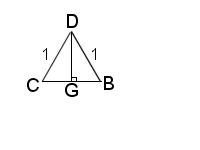Figure 4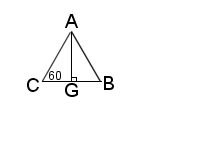Figure 5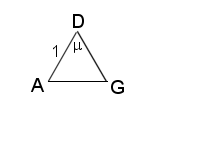Figure 6

Let us again bisect the angles λ, as shown in fig. 4.

$$BG = CG= \sin(\frac{\lambda}{2})$$, $$BC= 2\cdot \sin(\frac{\lambda}{2})$$, $$DG= \cos(\frac{\lambda}{2})$$.

Looking at the pyramid’s base ΔABC (fig. 5), $$BC = AC= AB = 2\cdot \sin(\frac{\lambda}{2})$$, then $$AG = BG\cdot\sqrt{3} = \sqrt{3}\cdot sin(\frac{\lambda}{2})$$.

We again us the law of cosines to find μ (fig. 6).

$$\Large\cos\mu=\frac{1+cos^{2}\frac{\lambda}{2} – 3\cdot sin^{2}\frac{\lambda}{2}}{2\cdot cos\frac{\lambda}{2}}$$

If we add $$(1\cdot sin^{2}\frac{\lambda}{2} -1\cdot sin^{2}\frac{\lambda}{2})$$, which equals zero, to the top of the equation, we get $$\large\cos\mu=\frac{1+(cos^{2}\frac{\lambda}{2}) – 4\cdot sin^{2}\frac{\lambda}{2}+(sin^{2}\frac{\lambda}{2})}{2\cdot cos\frac{\lambda}{2}}$$. Note the change of the three to four.

The items in parentheses can be combined using the identity cos² α + sin² α = 1:

$$\Large\cos\mu=\frac{1+1-4\cdot sin^{2}\frac{\lambda}{2}}{2\cdot cos\frac{\lambda}{2}}=\frac{2-4\cdot sin^{2}\frac{\lambda}{2}}{2\cdot cos\frac{\lambda}{2}}=\frac{1-2\cdot sin^{2}\frac{\lambda}{2}}{cos\frac{\lambda}{2}}$$.

Using the identity cos 2α = 1 – 2·sin² α, gives: $$\large\cos\mu=\frac{cos\lambda}{cos\frac{\lambda}{2}}$$.

We can then use this formula to verify the regular tetrahedron’s vertex edge angle that we found earlier. If $$\lambda=60^\circ$$, $$\large\cos\mu=\frac{cos60}{cos30}=\frac{\frac12}{\frac{\sqrt{3}}{2}}=\frac{1}{\sqrt{3}}$$, which is the same as θ above.

This formula can also be used on other polyhedra where there are three identical angles joining at a vertex, such as the cube,$$\large\cos\mu=\frac{cos90}{cos45}=\frac{0}{\frac{1}{\sqrt2}}=0, \mu=90^\circ$$ or dodecahedron, $$\large\cos\mu=\frac{cos108}{cos54}=\frac{\frac{1-\sqrt5}{4}}{\frac{\sqrt{10-2\sqrt5}}{4}}=\frac{1-\sqrt5}{\sqrt{10-2\sqrt5}}, \mu=121.71747^\circ$$.

I will get to the dodecahedron soon. The cube is so easy to figure out mathematically, rather boring.

Next posting, I will show you what happens if the pyramid leans a bit, that is if one of the apex angles is different that the other two.

# Finding the Angles of the Truncated Icosahedron

I’ve been interested in various geometric shapes for many years, from the simple cubes, prisms, and pyramids made in middle school, to the geodesic domes used to build houses.

I have cut and glued numerous pieces of paper into just about all of the regular polyhedra.

One project I have wanted to do for some time is to build a wire-frame truncated icosahedron, otherwise known as a buckyball or the general shape of a soccer ball. Folding paper and gluing the edges where they meet is a easy task, even for kids. Gluing wood dowels or soldering wire together at specific angles tends to be a wee bit more complicated, especially if you don’t know what the angles should be.

For any of the regular polyhedra, there are hundreds of internet sites and dozens of books that will detail out how to find the dihedral angle for each face-face edge. The trouble is, wire frames don’t have “faces,” each edge joins two or more edges at a point and spread out at equal or unequal angles that are never in the same plane. I have searched high and low, but have not yet found any website that gives these angles.

I have taken the painful steps of calculating these angles for the truncated icosahedron. Using these steps you can then find the angle for any of the other regular polyhedra.

First, let’s define a few items. The truncated icosahedron is made up of 12 pentagons and 20 hexagons, all with the same side length. Since they all have the same size sides, we will define every other measurement in terms of side length, and call it 1. It can be 1 inch, 1 cm, 1 mile, or 1 light-year, it doesn’t matter.

Each pentagon is surrounded by 5 hexagons. Each hexagon is surrounded by 3 pentagons and 3 hexagons, alternating. We will call each edge where a pentagon meets a hexagon a HP edge and where 2 hexagons meet, we’ll call a HH edge. Every edge will join with two others, always an HH edge and two HP edges will meet at each vertex.

The dihedral (face to face) angle for the HH edges is given as: $$\cos^{-1}\left(\Large{-\frac{\sqrt{5}}{3}}\right) = 138.189685\ldots^\circ$$.

The dihedral angle for the HP edges is: $$\cos^{-1}\left(\Large{-\sqrt{\frac{5+2\sqrt{5}} {15} } }\right) = 142.622632\ldots^\circ$$.

As I said, there are lots of sources to find the breakdown of the math used to get these results. I only add them here for your convenience.

The diameter can be “unrolled” to show there is a repeat (Fig 1) from P1 to P2 to P3. P3 would be the same point as P1.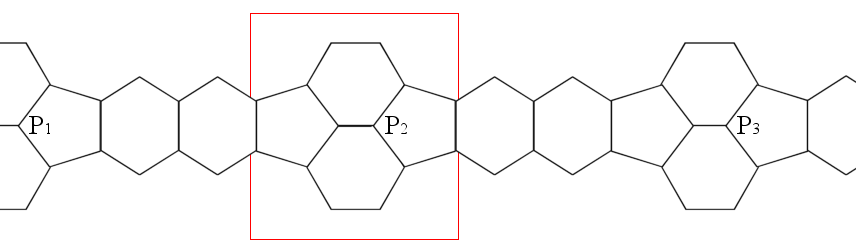This makes a decagon (10-sided figure), traveling across the faces of 4 pentagons, 4 hexagons, and 2 HH edges. This gives us a decagon with 4 HP angles and 2 HH angles, but we don’t know the other 4 Pentagon-to-Pentagon (Pent-Pent) edge angles.

All decagons have a total interior angle measurement of 1440°, using the formula for any N-gon, (N-2)x180. If we then use a little algebra, we get:

1440 = 4 * HP angle + 2 * HH angle + 4 * Pent-Pent edge angle

Substituting known values:

1440 = 4 x 142.622632 + 2 x 138.189685 + 4 x Pent-Pent edge angle
1440 = 570.490528 + 276.379370 + 4 x Pent-Pent edge angle
1440 = 846.869898 + 4 x Pent-Pent edge angle
1440 - 846.869898 = 4 x Pent-Pent edge angle
593.130102        = 4 x Pent-Pent edge angle
148.2825255       = Pent-Pent edge angle

So if I wish to create a wire-frame of a truncated icosahedron, then I just need to join two wires at 108°, the interior angle for a pentagon, and add a third sticking straight out and rising up at about an angle of 31¾° (180°-148¼°).

But, is this correct? How can it be verified? Is there a way to find “exact” measurements?

## Finding the Angles of a Pentagon-Hexagon-Hexagon Vertex

Taking the red-outlined area from Fig 1, and rotating we get Fig 2. Note, these figures are not to scale or proportion.

Drawing (blue) lines across the widths of the hexagons (KL & KM) and the pentagon (ML), we then get a pyramid with base ΔKLM and apex N (Fig 3).

Looking at ∠KNL and ∠KNM, each is 120° and ∠MNL=108°. The ΔNKL (Fig 4) shows how we get KL & KM from 30-60-90 triangles, each is $$2\cdot \sin60^\circ = 2·\frac{\sqrt3}{2} = \sqrt3$$.

ΔNML (Fig 5) shows the length for LM, we don’t need exact figures, 2·sin 54° will do for now. Lines GL & GM are sin 54°.

ΔKML (Fig 6) uses the Pythagorean Theorem to find length of line KG. We don’t need to find the actual length, as we are about to square it anyway.

ΔNKG (Fig 7) shows the angle η the we are looking for. Note: I drew this image even though knew that η was obtuse. 🙁

Using the Law of Cosines, we get:

\begin{align}\cos\eta &= \frac{1^2 + \color{Blue}{NG^2} – \color{LimeGreen}{KG^2}}{2\cdot1\cdot \color{Blue}{NG}}\\ &= \frac{1 + \color{Blue}{cos^2(54)} – \color{LimeGreen}{\bigl [4\cdot sin^2(60) – sin^2(54)\bigr ]}}{2\cdot1\cdot \color{Blue}{cos (54)}}\\ &= \frac{1 + \color{Blue}{cos^2(54)} \color{LimeGreen}{- 4\cdot sin^2(60)} + \color{LimeGreen}{sin^2(54)}}{2\cdot \color{Blue}{cos (54)}}\\ &= \frac{1 + \color{red}[\color{Blue}{cos^2(54)} + \color{LimeGreen}{sin^2(54)}\color{red}] \color{LimeGreen}{- 4\cdot sin^2(60)}}{2\cdot \color{Blue}{cos (54)}} & \text{Rearrange}\\ &= \frac{1 + \color{red}1 – 4\cdot sin^2(60)}{2\cdot \color{Blue}{cos (54)}} & \text{Using the identity cos² α + sin² α = 1}\\ &= \frac{2 – 4\cdot sin^2(60)}{2\cdot \color{Blue}{cos (54)}}\\ &= \frac{\color{yellow}{1 – 2\cdot sin^2(60)}}{\color{Blue}{cos (54)}} & \text{factor out 2}\\ &= \frac{\color{yellow}{\cos(2\cdot 60)}}{\color{Blue}{cos (54)}} & \text{Using the identity cos 2α = 1 – 2·sin² α}\\ &= \frac{\color{yellow}{cos(120)}}{\color{Blue}{cos(54)}}\\ &= \frac{-\frac12} {\sqrt{\frac{5-\sqrt{5}} {8} } }\\ &= \frac{-1}{2} \cdot \frac{\sqrt{8}}{\sqrt{5-\sqrt{5}}} & \text{invert}\\ &= \frac{-2\sqrt2}{2\sqrt{5-\sqrt{5}}}\cdot \frac{\sqrt{5+\sqrt{5}}}{\sqrt{5+\sqrt{5}}} & \text{Multiply by conjugate}\\ &= \frac{-\sqrt2\sqrt{5+\sqrt{5}}}{\sqrt{25-5}}\\ &= \frac{-\sqrt2\sqrt{5+\sqrt{5}}}{\sqrt{20}}\\ &= \frac{-\sqrt2\sqrt{5+\sqrt{5}}}{\sqrt{4\cdot 5}}\\ &= \frac{-\sqrt2\sqrt{5+\sqrt{5}}}{2\sqrt5}\\ &= \frac{-\sqrt2\sqrt{5+\sqrt{5}}}{2\sqrt5}\cdot \frac{\sqrt5}{\sqrt5}\\ &= \frac{-\sqrt2\sqrt5\sqrt{5+\sqrt{5}}}{20}\\ &= -\sqrt{\frac{5+\sqrt5}{10} } \end{align}
∠η = 148.2825255…°

Hooray! It matches the value we found earlier.

## Finding the Angles of a Regular Pentagonal Pyramid

A truncated icosahedron is made by truncating (cutting off) a regular pentagonal pyramid from each of the tips of an icosahedron, hence the name.

The angle of the pentagon face to any of the triangle edges will be 180° minus the Pent-Pent edge angle.

In order to find the angles we want, we must first find the height of a pentagon (Fig 8a). Either use the Pythagorean Theorem or trigonometry to find height H.

The faces of the pyramid are all equilateral triangles. Finding the triangle height S is elementary (Fig 8b). Side note: S is 1/3 the length of the original icosahedron.

Slicing through the pyramid through points A, B, and C, reveals (Fig 8c) ΔABC.

We then use the Law of Cosines to find ∠θ.

\begin{align}\cos \theta &= \frac{1^2 + \color{skyblue}{H^2} – \color{limegreen}{S^2}}{2\cdot1\cdot \color{skyblue}H}\\ &= \frac{1^2 + \color{skyblue}{\frac14\cdot tan^2(72)} \color{limegreen}{-\frac34}}{2\cdot1\cdot \color{skyblue}{\frac12\cdot tan(72)}}\\ &= \frac{\frac44 \color{limegreen}{-\frac34} + \color{skyblue}{\frac14\cdot(5+2\sqrt{5})} }{2\cdot \color{skyblue}{\frac12\sqrt{5+2\sqrt{5}}}} \\ &= \frac{\frac14+\frac14(5+2\sqrt5)}{\sqrt{5+2\sqrt5}} \\ &= \frac{1+\quad 5+2\sqrt5}{4\sqrt{5+2\sqrt5}}\\ &= \frac{6+2\sqrt5}{4\sqrt{5+2\sqrt5}}\\ &=\frac{3 + \sqrt{5}} {2\sqrt{5 + 2\sqrt{5}}}\\ &=\frac{3 + \sqrt{5}} {2\sqrt{5 + 2\sqrt{5}}} \cdot \frac{\sqrt{5 – 2\sqrt{5}}}{\sqrt{5 – 2\sqrt{5}}} & \text{multiply by conjugate}\\ &=\frac{(3 + \sqrt{5})\sqrt{5-2\sqrt5}} {2\sqrt{25-20}} \\ &=\frac{\color{yellow}{(3 + \sqrt{5})}\sqrt{5-2\sqrt5}} {2\sqrt5} \\ &=\frac{\color{yellow}{\sqrt{14 + 6\sqrt{5}}}\sqrt{5-2\sqrt5}} {2\sqrt5} & \color{yellow}{(3 + \sqrt{5})^2 = 14+6\sqrt5}\\ &=\frac{\sqrt{70 – 60 + 30\sqrt5 -28\sqrt5}} {2\sqrt5} \\ &=\frac{\sqrt{10 + 2\sqrt5}} {2\sqrt5} \\ &=\frac{\sqrt{10 + 2\sqrt5}} {2\sqrt5} \cdot \frac{\sqrt5}{\sqrt5} \\ &=\frac{\sqrt{50 + 10\sqrt5}} {10} \\ &=\frac{\sqrt{10}\sqrt{5 + \sqrt5}} {10} \\ &=\sqrt{\frac{5 + \sqrt5}{10}}\end{align}

∠θ = 31.7174744…°

θ + η = 180°

$$\cos \theta = -\cos \mu$$